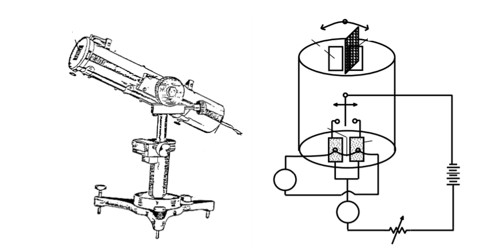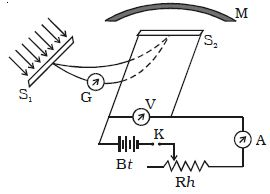# Define and Discuss on Angstrom Pyrheliometer

Pyrheliometer is an instrument used to measure the quantity of heat radiation and solar constant. It is a type of actinometer that can measure solar irradiance in the desired location and solar radiation flux density.

Pyrheliometer designed by Angstrom is the simplest and most accurate. This is a dependable instrument used to monitor direct solar radiation and has long been accepted as a working standard. The solar radiation spectrum extends approximately between 300 and 2800 nm.

Working principle:

A pyranometer is operated based on the measurement of the temperature difference between a clear surface and a dark surface. The black coating on the thermopile sensor absorbs the solar radiation, while the clear surface reflects it, and hence less heat is absorbed. A pyrheliometer is used to measure direct solar radiation from the sun and its marginal periphery.

Angstrom’s pyrheliometer consists of two identical strips S1 and S2 of area A. One junction of a thermocouple is connected to S1 and the other junction is connected to S2. A sensitive galvanometer is connected to the thermocouple.

Strip S2 is connected to an external electrical circuit as shown in Figure.When both the strips S1 and S2 are shielded from the solar radiation, the galvanometer shows no deflection as both the junctions are at the same temperature. Now strip S1 is exposed to the solar radiation and S2 is shielded with a cover M. As strip Si receives heat radiations from the sun, its temperature rises and hence the galvanometer shows deflection. Now current is allowed to pass through the strip S2 and it is adjusted so that the galvanometer shows no deflection. Now, the strips S1 and S2 are again at the same temperature.

If the quantity of heat radiation that is incident on the unit area in unit time on strip S1 is Q and its absorption coefficient, then the amount of heat radiations absorbed by the strip S1 in unit time is QAa.

Also, the heat produced in unit time in the strip; is given by VI, where V is the potential difference and I is the current flowing through it.

As heat absorbed = heat produced

QAa = VI or Q = VI/Aa

Knowing the value of V, I, A and a, Q can be calculated.

• Very little temperature coefficient,
• Calibrated to ISO standards,
• More perfect measurements of performance index and performance ratio
• Longer response time than a photovoltaic cell

Applications

• Predicting insulation requirements for building structures,
• Establishment of greenhouse locations,
• Designing photovoltaic systems,
• Measurement of solar intensity data.